# Create your own formulas: use the correct JavaScript syntax for each type of calculated element

With AODocs, you can automatically calculate the following elements in your documents:

Note: Calculated settings are currently in private beta. To request access to the private beta, reach out to us: aodocs-beta@aodocs.com.

These elements can be calculated using formulas that can include references to other elements, thereby creating dependencies between your properties, settings and titles. Library administrators can create calculated elements in any type of library.

This article explains the JavaScript syntax you can use in each type of calculated element. It also provides examples of the corresponding calculated values you can expect.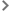Note about different types of JavaScript syntaxCalculated String or Text properties and calculated titlesCalculated Integer propertiesCalculated Decimal propertiesCalculated Boolean properties and calculated settingsCalculated Date, Date&Time or Time propertiesCalculated Category propertiesCalculated Person propertiesCalculated URL properties

## Note about different types of JavaScript syntax

In the examples we give in our articles about calculated elements (learn more: Getting started with formulas for calculated elements), we use two types of JavaScript syntax:

• single expression
• multiple instructions, wrapped in an arrow function

You can use either in your calculated properties, but we recommended taking account of the following considerations.

General rule: the JavaScript code defining a formula must be made of one, and only one, expression.

This means that:

• if your logic can be expressed in a single expression, you can type it directly in the formula builder, for example:

`property`Project name`+' - '+sequenceId`document``

• if your code requires additional instructions (such as when processing dates), you should wrap all your statements in an arrow function definition `(() => { ... })`, and call it right after the definition with an arrow function call `()`:

``` (() => { const d=new Date(property`Publication date`) const yyyy=d.getFullYear(), const mm=`\${d.getMonth()+1}`. padStart(2,'0') const dd=`\${d.getDate()}`.padStart( 2,'0') return `\${mm}/\${dd}/\${yyyy}` })() ```

However, you can play with JavaScript to combine these multiple instructions into a single expression on a single line:

``` `\${`\${(new Date(property`Publication date`)) .getMonth()+1}`. padStart(2,'0') }/\${`\${(new Date(property`Publication date`)) .getDate()}`.padStart( 2,'0') }/\${(new Date(property`Publication date`)).getFullYear()}`  ```

You can then enter this directly in the formula builder without using the arrow function definition.

We recommend using the arrow function definition because with the single-line expression:

• there's a lot of repetition, which is not good practice; in our example ```new Date(property`Publication date`)``` is repeated three times
• the expression is long, which makes it difficult to read and debug

## Calculated String or Text properties and calculated titles

String and Text properties, and also calculated titles, accept as input:

• Strings
• Integers
• Decimals
• Booleans

They also support simple math calculations, which are evaluated when the formula is calculated and the result of which is displayed. Learn more: Use simple math calculations.

Below are some supported JavaScript expressions with their expected values.

 JavaScript expression JavaScript type Value of calculated property or title Comment `'Document validated'` StringValue: `Document validated` Single quotes `"Document validated"` String Value: `Document validated` Double quotes `1234` Number Value: `1234` No formatting `3.0` Number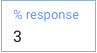Value: `3` No formatting `1+2` Number Value: `3` Evaluation first `true` or`false` Boolean Value: `true` or`false` English ``\${3+4} documents`` String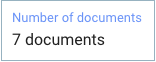Value: `7 documents` String literal

## Calculated Integer properties

The nominal expected value is a JavaScript Number, but a best effort is made to also map String types.

Below are some supported JavaScript expressions with their expected integer values.

 JavaScript expression JavaScript type Value of calculated property Comment `42` Number Value: `42` Nominal integer `1+2` Number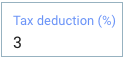Value: `3` Operation `5.0` Number Value: `5` No decimal part `3.14` Number Value: `3` Round-down `3.8` Number Value: `3` Round-down `'12'` String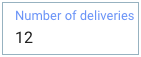Value: `12` String parsing integer `"8.2"` String Value: `8` String parsing decimal

## Calculated Decimal properties

The nominal expected value is a JavaScript Number, but a best effort is made to also map String types.

Below are some supported JavaScript expressions with their expected decimal values.

 JavaScript expression JavaScript type Value of calculated property Comment 3.14 Number Value: `3.14 ` Nominal decimal 1.2+3.8 Number Value: 5 Operation 4 Number Value: 4 Integer 2 + 3.1 Number Value: `5.1 ` Hybrid operation ‘6.626’ String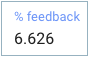Value: `6.626` String parsing decimal 1 + 0.61 Number Value: `1.61 `(not 1.6099999999999999) Double precision:16 bits

## Calculated Boolean properties and calculated settings

For Boolean properties and calculated settings, the nominal expected value is a JavaScript Boolean, but a best effort is made to also map String types.

Below are some supported JavaScript expressions with their expected boolean values.

 JavaScript expression JavaScript type Value of calculated property or setting Comment `true` or `false` BooleanValue:`true` or `false`(in this example, `true`) Nominal `‘true’` or `‘false’` StringValue:`true` or `false`(in this example, `false`) String in english `‘TRUE’` or `‘FALSE’` String Value: `true` or `false` String in ENGLISH

## Calculated Date, Date&Time or Time properties

The nominal expected values are either JavaScript Date or Number. Strings are not supported.

Notes:
– Dates and times are given in Epoch milliseconds (the number of milliseconds since January 1, 1970, 00:00:00 UTC).
– Months in JavaScript start at zero: January is 0, February is 1, and so on. Learn more: Extract the month.
–  Date properties must use a value that corresponds to midnight UTC, otherwise an error occurs.
– The display of dates varies according to the user's locale. For example, en_GB, en_US and fr_FR all display dates differently.

Below are some supported JavaScript expressions with their expected Date values.

 JavaScript expression JavaScript type Value of calculated property Comment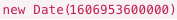Date AODocs Date property:JavaScript Date `483211100000` Number AODocs Date&Time property: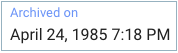Value: `April 24, 1985 7:18 PM` AODocs translates the number into a JavaScript Date `1607544327000` Number AODocs Time property: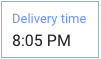Value: `8:05 PM` AODocs translates the number into a JavaScript Date `new Date(2015,3,1)` Date AODocs Date&Time property: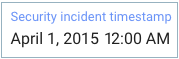Value: `April 1, 2015 12:00 AM` Note: If you don't indicate time in the code, the time defaults to 12 AM UTC (or 12 AM UTC plus however many hours ahead of UTC your timezone is). JavaScript Date `new Date(2015,3,1,10,30)` Date AODocs Date&Time property: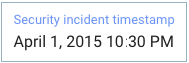Value: `April 1, 2015 10:30 PM` JavaScript Date

## Calculated Category properties

The nominal expected value is a String equal to the selected category value ID

 JavaScript expression Structure of the category value entity reference:#{categoryValue:category_ID/category_value_ID} For example: `'#{categoryValue:SGb0BhbtJUsWGRDo3z/SGb2pVav2f0yuuwpp9}'` Important: Use the code injector in the Formula pop-up. Learn more: Create calculated properties. JavaScript type String Value of calculated Category property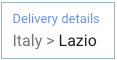Value: `Italy > Lazio`

## Calculated Person properties

The nominal expected value is a String equal to the email address of to the person or group you are referring to.

 JavaScript expression Email address. For example: alice@demokb.aodocs.com JavaScript type String Value of calculated Person property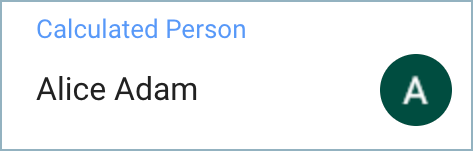Value: `alice@demokb.aodocs.com`

## Calculated URL properties

The nominal expected value is a String. In its simplest form, this value is equal to the web address. It's also possible to provide a label that is displayed instead of the address. You can use the syntax: `[label](address)`.

Below are some supported JavaScript expressions with their expected URL values.

 JavaScript expression JavaScript type Value of calculated property Comment `https://www.aodocs.com` String AODocs simple URL property: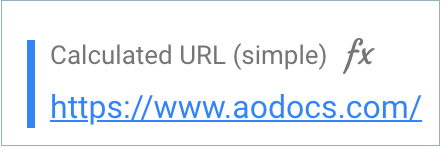The raw address is displayed in the AODocs document `[AODocs website](https://www.aodocs.com)` String AODocs URL property with label: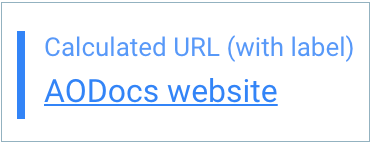The label is displayed in the AODocs document